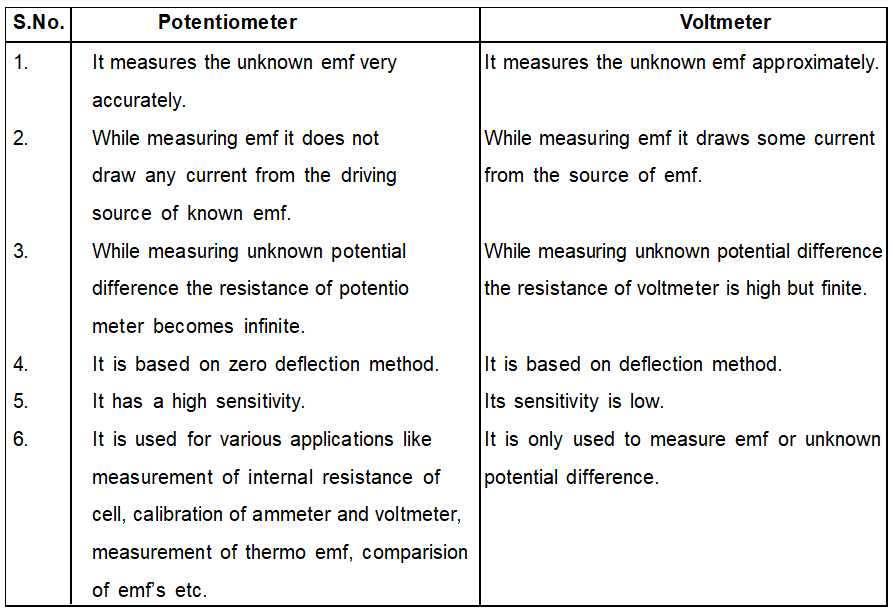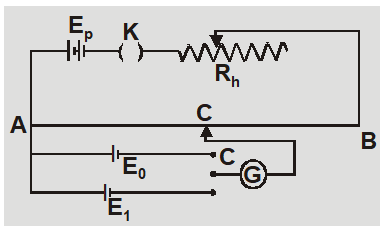Download the eSaral app and start learning from Kota's top IITians and doctors.

# Difference Between Potentiometer and Voltmeter - Current Electricity || Class 12, JEE & NEET

The Potentiometer and the Voltmeter both are the voltage measuring devices. The main Difference Between Potentiometer and Voltmeteris that the potentiometer measures the emf of the circuit whereas voltmeter measures the end terminal voltage of the circuit. The other differences between the Potentiometer and the voltmeter are explained below in the comparison chart.

### Comparison Chart## Standardization of Potentiometer

The process of determination of potential gradient on wire of potentiometer is known as standardisation of potentiometer. A standard cell is one whose emf remains constant. Cadmium cell with emf 1.0186 V at $20^{\circ}$C is used as a standard cell. In laboratory a Daniel cell with emf 1.08 V is usually used as a standard cell.If $\ell_{0}$ is the balancing length for standard emf $E_{0}$ then potential gradient $x=\frac{E_{0}}{l_{0}}$

### Key Differences:

The following are the key differences between Potentiometer and voltmeter.
1. The Potentiometer is an instrument used for measuring the emf, whereas the voltmeter is a type of meter which measures the terminal voltage of the circuit.
2. The Potentiometer accurately measures the potential difference because of zero internal resistance. Whereas, the voltmeter has a high internal resistance which causes the error in measurement. Thus the voltmeter approximately measures the voltage.
3. The sensitivity of the Potentiometer is very high, i.e. it can measure small potential differences between the two points. The voltmeter has low sensitivity.
4. The Potentiometer uses the deflection type instrument whereas the voltmeter uses the deflection type instrument.
5. The Potentiometer has infinite internal resistance, whereas the Potentiometer has high measurable resistance.
Watch out the Video: Applications of Potentiometer & its Construction by Saransh Sir.

### Conclusion

The Potentiometer and voltmeter both measures the emf in volts. The Potentiometer is used in a circuit where the accurate value of voltage is required. For approximate calculation, the voltmeter is used.   Physics Revision Series by Saransh Sir (AIR 41 in IIT-JEE)